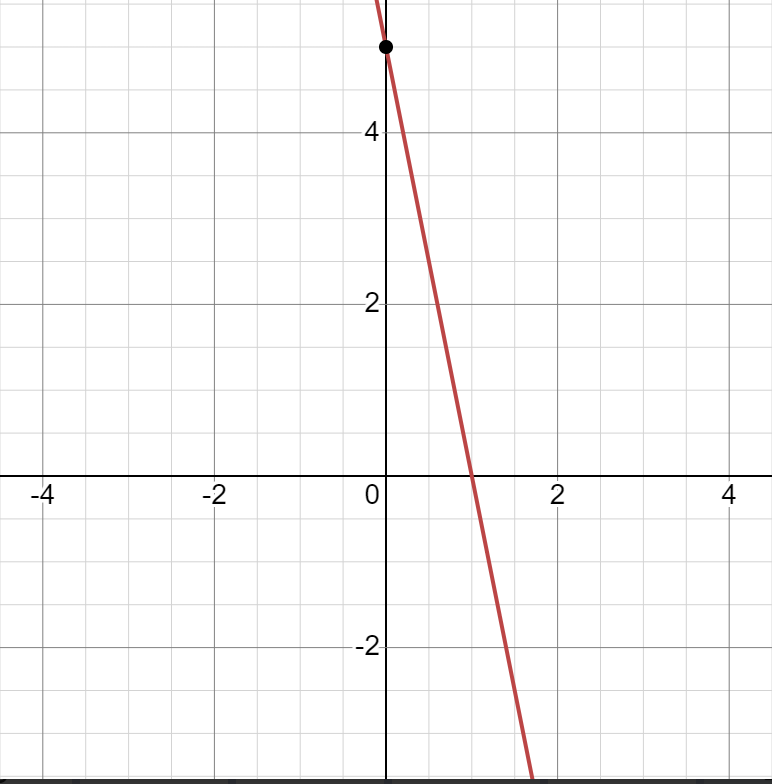# Find then slope and y-intercept of the line. Graph theAnish Buchanan 2021-08-15 Answered
Find then slope and y-intercept of the line. Graph the line.
$$\displaystyle{5}{x}+{y}={5}$$

• Questions are typically answered in as fast as 30 minutes

### Plainmath recommends

• Get a detailed answer even on the hardest topics.
• Ask an expert for a step-by-step guidance to learn to do it yourself.Dora

Let's rewrite it as an equation with a slope.
Slope equation type:
$$\displaystyle{y}={m}{x}+{b}$$, where equals the slope and b equals the y-intercept of the line.
Subtract 5x from both sides of the equation.
$$\displaystyle{y}={5}-{5}{x}$$
Replace 5 and -5x.
Let's use the equation for a bunch of straight lines passing through a given point in order to find the slope and the point of intersection with the y-axis.
Find the values ​​of m and b using the form
$$\displaystyle{y}={m}{x}+{b}$$
$$\displaystyle{m}=-{5}$$
$$\displaystyle{b}={5}$$
The slope of the line is m, and the y-intercept is b.
Slope: −5
y-intercept: (0, 5)